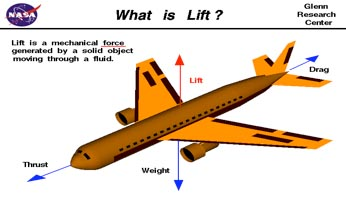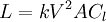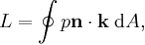# Lift

## Lift

Lift is one of four forces that create flight. It is directly related to temperature, pressure, and air density. If the air is colder, then the air density is higher and so there is more air to create lift. Likewise, if the air is warmer, then it is also less dense and less likely to create lift. The pressure of the air around the aircraft is not only determined by the atmosphere but also by the velocity of the plane. Lift, by nature, is the pressure difference of air forcing the wings of the plane upwards and thus forcing the plane itself upwards. The counterpart of lift is weight. Weight is the force of gravity acting down on the plane. The other two forces are thrust and drag. Thrust is the force of the propeller moving air through it, pulling the plane forward, and drag, its counterpart, is the force of the air pushing against the plane. In straight and level, unaccelerated flight, lift = weight and thrust = drag. However, in order for lift to be in equilibrium with weight, the plane can be neither climbing nor descending.The lift operates mainly by Bernoulli’s principle. It does so with the help of an airfoil (airplane wing) moving forward and compressing the air in front of it. This is where Bernoulli’s principle comes into play. The angle of the airfoil relative to the direction of the air moving past it (also known as the angle of attack) is higher, so the top of the wing experiences less air compression than the bottom of the wing. Since the air is being compressed more on the bottom than on the top, its velocity decreases. This means that the velocity of the air over the top of the wing is higher since its pressure is lower. Bernoulli states that an area of high pressure will always move to an area of low pressure, and so a higher air pressure on the bottom of the wing forces it up and thus forces the airplane to fly.

A little confusing at first to understand, so let's use the example of a garden hose. There cannot be more water going into the hose than there is leaving. If there could, then the hose would start to blow up in the middle like a balloon. If you put your finger over the end of the hose, the water shoots out faster. This is because the same amount of water is entering the hose, but the hole in which it’s leaving is smaller. The pressure of the water behind your finger becomes higher, and so the velocity of the water going into the hose decreases. The pressure of the water on the outside of your finger is lower and so the velocity becomes faster, making the water shoot at a faster speed.

There are a few equations that represent lift. The first was developed by John Smeaton and used by the Wright brothers in 1908.Where:

• L is the lift,
• k is the Smeaton coefficient- 0.005 (the drag of a 1-square-foot (0.093 m2) plate at 1 mph),
• Cl is the lift coefficient (the lift relative to the/or L/D),
• A is the area in square feet.

As aviation progressed, the understanding of lift became more necessary and so the equation became more complex. Bernoulli’s principle helped determine how much lift was being produced for a specific sized wing, and so the following equation was developed:Where:

• L = the lift,
• A = the wing surface area,
• p = the value of the pressure,
• n = the normal unit vector pointing into the wing,
• k = the vertical unit vector, normal to the free stream direction.

## Get a Quote for Your trip

•••••••••